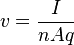# Drift Velocity in a Vaccum Diode

Gold Member
Is the standard equation for drift velocity of electrons also applicable to conduction in, say, a vacuum tube?I assume it is, and if so what is the relation between the drift velocities at the interface of vacuum-metal at the anode of a vacuum diode? The current, electronic charge and Area remain the same so I suppose it is only the ratio of the carrier density? (Let's assume we are operating the diode in its saturation current region)

The conductor density in a metal is fixed (say, 8.5×1028 electrons per m³ for Copper) but what determines the density in the vacuum (again, in the saturation region)?

Beyond saturation, even on a bias voltage increase the current remains the same. The explanations I've read say this is because all the electrons emitted by thermionic emission have been used up. But wouldn't it be possible to increase current via an increase in their drift velocity? Wouldn't increasing voltage increase the field thereby accelerating electrons ultimately increasing drift velocities?

Why does current saturate then?

Last edited:

## Answers and Replies

Gold Member
But wouldn't it be possible to increase current via an increase in their drift velocity? Wouldn't increasing voltage increase the field thereby accelerating electrons ultimately increasing drift velocities?

That argument doesn't work. If the cathode can only produce a certain number of electrons per second, that is the limit to the current. Making them go faster will (after the steady sate has been reached) just mean they hit the anode with higher energy (i.e. more Power in and out)

Gold Member
That argument doesn't work. If the cathode can only produce a certain number of electrons per second, that is the limit to the current. Making them go faster will (after the steady sate has been reached) just mean they hit the anode with higher energy (i.e. more Power in and out)

Thanks! But how does that tie in with this equation?The current seems proportional to velocity?

Higher energy, like you said they would have, is Kinetic Energy, I assume? If so the velocity increases? Increasing current by the equation above? What gives?

Of course, experiment tells you are right, I'm only trying to round off my understanding!

Gold Member
I do see what you mean though. In a I = Q/t sense if the Q/t reaches its limit I cannot increase any more.

I just cannot see the consistency with the drift velocity equation.

Gold Member
Here's why. If they move faster. There is more space between them so the density goes down accordingly.
Simples.

Gold Member
Here's why. If they move faster. There is more space between them so the density goes down accordingly.
Simples.

Makes sense! Thanks.

So till the saturation point density remains fairly constant, only velocity rises? Or is it some combination of both factors.

Gold Member
Here's why. If they move faster. There is more space between them so the density goes down accordingly.
Simples.

The other mystery is why does compensation happen in a way that keeps velocity x density constant?

Otherwise current wouldn't stay saturated?

Gold Member
Space between electrons is proportional to speed- all the time, with a lower limit set by the mutual repulsion, I guess.

One of the effects we see (in microelectronic engineering) with high density ion currents in vacuum and low energy is beam "blowup" due to space charge.

http://en.wikipedia.org/wiki/Space_charge
http://www.casetechnology.com/implanter/neutral.html

Gold Member
One of the effects we see (in microelectronic engineering) with high density ion currents in vacuum and low energy is beam "blowup" due to space charge.

http://en.wikipedia.org/wiki/Space_charge
http://www.casetechnology.com/implanter/neutral.html

And hence the need to focus the beam in CRTs and other electron beam devices. In a diode, however, it's a bit harder to foresee what'll happen because the cathode is often along the axis of a cylindrical anode. Perhaps the electrons are 'squished' out of the ends of the cylinder due to the repulsion when low HT is used. It's been so long since I used a thermionic diode. . . .

Gold Member
And hence the need to focus the beam in CRTs and other electron beam devices. In a diode, however, it's a bit harder to foresee what'll happen because the cathode is often along the axis of a cylindrical anode. Perhaps the electrons are 'squished' out of the ends of the cylinder due to the repulsion when low HT is used. It's been so long since I used a thermionic diode. . . .

Actually, what got me thinking about this was saturation currents in the photoelectric effect.

But guess it's a similar phenomenon. Carrier depletion compensates for KE increases.